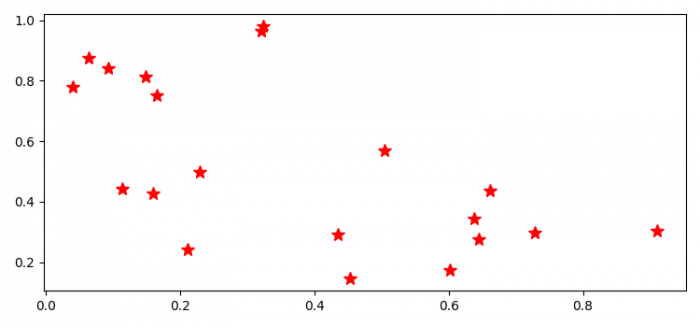# How can I make Matplotlib.pyplot stop forcing the style of my markers?

To make matplotlib.pyplot stop forcing the style of markers, we can take the following steps −

• Set the figure size and adjust the padding between and around the subplots.
• Create random x and y data points using numpy.
• Plot x and y data points using plot() method, with "r*" marker with markersize=10.
• To display the figure, use show() method.

## Example

from matplotlib import pyplot as plt
import numpy as np

plt.rcParams["figure.figsize"] = [7.50, 3.50]
plt.rcParams["figure.autolayout"] = True

x = np.random.rand(20)
y = np.random.rand(20)

plt.plot(x, y, 'r*', markersize=10)

plt.show()

## Output